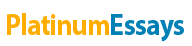# In Answering the Questions, You Should Supply Easy-To-Follow Calculations And/or Reasoning and Not Simply Write Down an Answer.

By:   •  October 20, 2014  •  Essay  •  791 Words (4 Pages)  •  1,097 Views

Page 1 of 4

Note: In answering the questions, you should supply easy-to-follow calculations and/or reasoning and not simply write down an answer.

This homework concerns the Orion Bus Company case in your course packet (or in BlackBoard) and the associated dataset ("Orion Bus Bids.xlsx") on Orion's recent bids. Read the case. Our objective is to develop a statistical model to enable Orion to improve its bidding. The dataset has data on a sample of 41 Orion bids that the company lost. The winning bid on each contract is "LowBid", which is the lowest bid of Orion's competitors. Orion's bid is not shown. But Orion's estimate of the cost to build per bus is shown ("OrionsEstimatedCost"). Also shown are some contractual specifications: the number of buses in each contract, the length of the bus in feet, whether the bus was to be diesel or not (1=Yes, 0-No), whether the bus was to be a high-floor bus or not (1=Yes, 0=No). Finally, an indicator (US) for whether the bid requestor was located in the United States (1) or Canada (0).

1. Orion management argues that it is appropriate to analyze only bids that Orion lost. As reasons, Orion management says that the winning bids in these cases were competitor's bids. Orion lost out on these contracts. Orion would like to profit from analysis of competitors' winning bids in order to improve its own chances of winning some of these. Study of Orion's winning bids would not give it that information. What do you think? Should Orion analyze only the contracts that it lost?

Run the following regression: Y=LowBid, X1= NumberOfBusesInContract, X2= OrionsEstimatedCost, X3=Length. Assume LHIN.

2. Suppose that Orion is planning to submit a bid on a contract to supply the City of Louisville, Kentucky, U.S.A., with 5 buses that would each be 30-feet long, and that Orion estimates to cost \$234,229 per bus to build. Estimate the winning bid of Orion's competitors.

3. You can reasonably expect the actual winning bid of Orion's competitors to differ from your estimate in the preceding question by about ±\$_______________. How much confidence can you have that the actual winning bid will be within that much of your estimate?

There is uncertainty about what the winning bid of Orion's competitors on the Louisville contract will be. For the remaining questions, suppose that an adequate representation of that uncertainty is a normal distribution having a mean equal to your estimate in question 2 and a standard deviation equal to the St Err of Estimate in your regression model. Also assume that Orion's actual cost will equal its estimate for any contract.

4. Suppose that Orion bids the mean of the winning bid uncertainty distribution. If Orion wins the contract, then Orion's gross profit per bus will be \$________.

5. Suppose that Orion bids the mean of the winning bid uncertainty distribution. The probability that Orion will win the contract is _____________. [Hint: Orion wins if its competitors' LowBid exceeds Orion's bid.]

Let X = Orion's profit per bus. There is uncertainty about the value that X will be.

6. The set all possible values of X is [list them]_______________. [Hint: Either Orion wins or loses.]

7. The corresponding probabilities for each possible value of X are ______________.

8. The expected value (mean) of X is _________________.

9. Using the preceding questions as guides, fill in the following table. [Hint: Use Excel, fill in the first row, and copy your formulas down the rows.]

If Orion bids this column … and wins, then its profit per bus will be … Orion's probability of winning

...